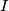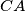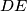### IMO Shortlist 1993 problem G1

Kvaliteta:
Avg: 0,0
Težina:
Avg: 6,0
Let$ABC$ be a triangle, and$I$ its incenter. Consider a circle which lies inside the circumcircle of triangle$ABC$ and touches it, and which also touches the sides$CA$ and$BC$ of triangle$ABC$ at the points$D$ and$E$, respectively. Show that the point$I$ is the midpoint of the segment$DE$.
Izvor: Međunarodna matematička olimpijada, shortlist 1993